# Identify the conjugate acid for cach base. conjugate acid of HCO : conjugate acid of HPO...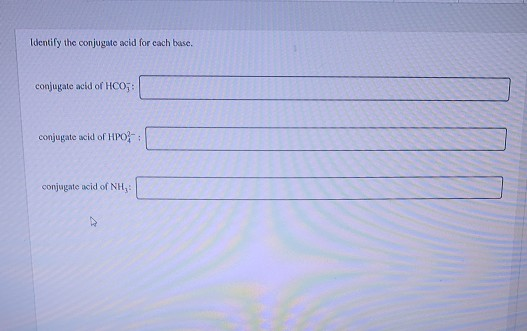Identify the conjugate acid for cach base. conjugate acid of HCO : conjugate acid of HPO conjugate acid of NH,

the conjugate acid of some ion is a compound that formed after addition of proton. thus conjugate acid of HCO3- is H+ + HCO3- = H2CO3 ;

as same as for the conugate acid of HPO42- = H+ + HPO42- = H2PO4- and the conjugate acid of NH3 = H+ + NH3 = NH4+

##### Add Answer of: Identify the conjugate acid for cach base. conjugate acid of HCO : conjugate acid of HPO...
Similar Homework Help Questions
• ### identify the conjugate acid of hco-3

identify the conjugate acid of hco-3

• ### Identify the conjugate base for each acid. conjugate base of H,PO, conjugate base of H, PO...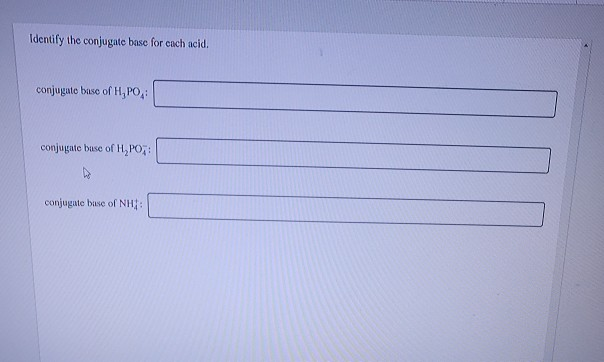Identify the conjugate base for each acid. conjugate base of H,PO, conjugate base of H, PO conjugate base of NH:

• ### stion 8 of 14 > Attempt 1 Identify the conjugate acid for each base. conjugate acid...stion 8 of 14 > Attempt 1 Identify the conjugate acid for each base. conjugate acid of HS : H,S conjugate acid of PO H SO- conjugate acid of NH, NH,+ EN ..

• ### Problems Identify the acid, base, conjugate acid, and conjugate base in the following reactions. (4 pts....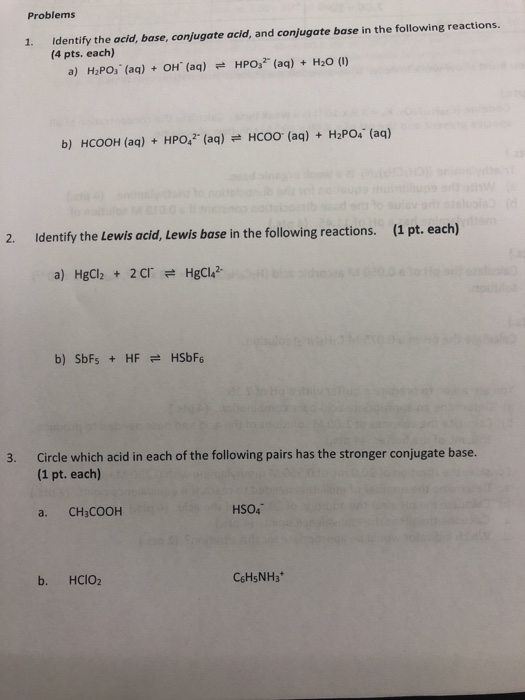Problems Identify the acid, base, conjugate acid, and conjugate base in the following reactions. (4 pts. each) 1. a) H2PO3 (aq) OH (aq) HPO32 (aq) H2O (I) HCOO (aq) H2Po4 (aq) b) HCOOH (ag) + HPO,2 (aq) 2. Identify the Lewis acid, Lewis base in the following reactions. (1 pt. each) a) HgCh 2CT HgCl? b) SbFs HFHSbF6 Circle which acid in each of the following pairs has the stronger conjugate base. (1 pt. each) 3. a. CH3COOH HSO4 b....

• ### acid base conjugate acid conjugale base HPO 2- (aq) + H20(1) = H,PO, - (aq) +...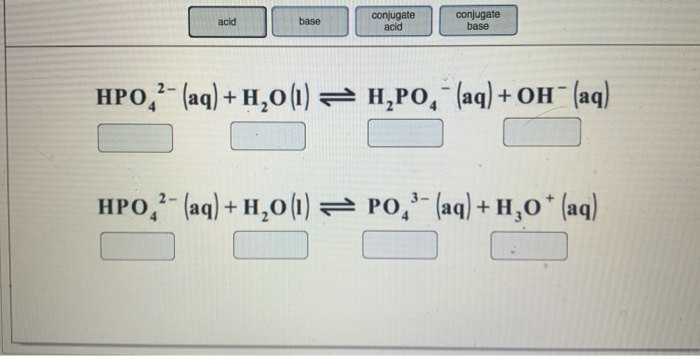acid base conjugate acid conjugale base HPO 2- (aq) + H20(1) = H,PO, - (aq) + OH" (aq) HPO,?- (aq) + H20(1) = PO,'- (aq) + H,0* (aq)

• ### 3. (a) Identify the acid & it's conjugate base and the base & it's conjugate acid...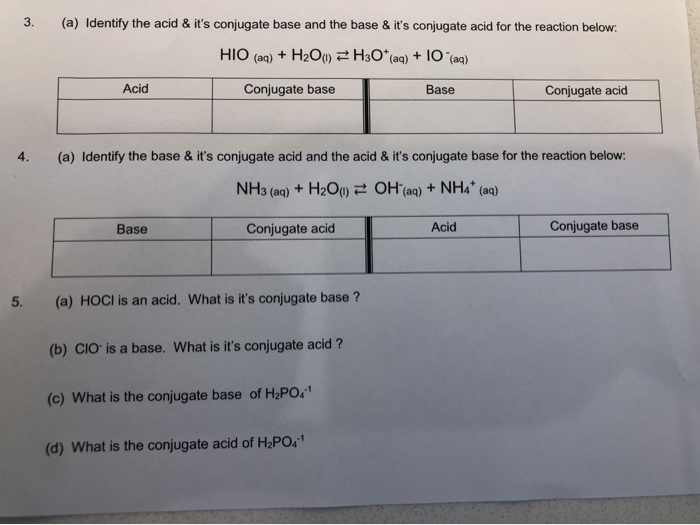3. (a) Identify the acid & it's conjugate base and the base & it's conjugate acid for the reaction below. HIO (aq) + H2O() H3O+ (aq) + 10 (aq) Acid Conjugate base Base Conjugate acid 4. (a) Identify the base & it's conjugate acid and the acid & it's conjugate base for the reaction below: NH3 (aq) + H2O) = OH(aq) + NH4* (aq) Base Conjugate acid Acid Conjugate base 5. (a) HOCI is an acid. What is it's conjugate...

• ### Which of the following are conjugate acid base pairs? 1. CO2 and HCO, 2. HCIO4 and...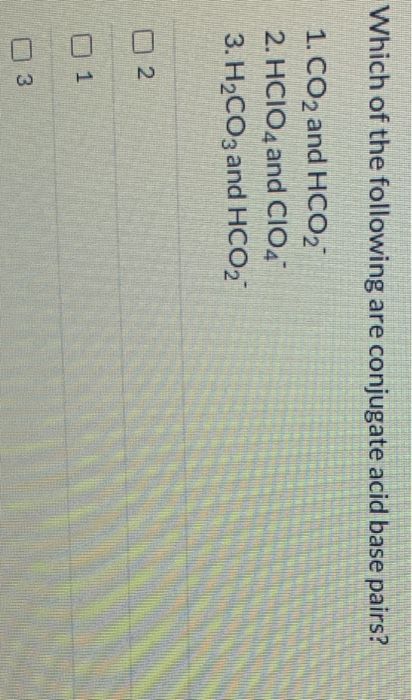Which of the following are conjugate acid base pairs? 1. CO2 and HCO, 2. HCIO4 and CIO4 3. H2CO3 and HCO, 2 3

• ### 1. Identify the acid (A), base (B), conjugate acid (CA) and the conjugate base (CB) in...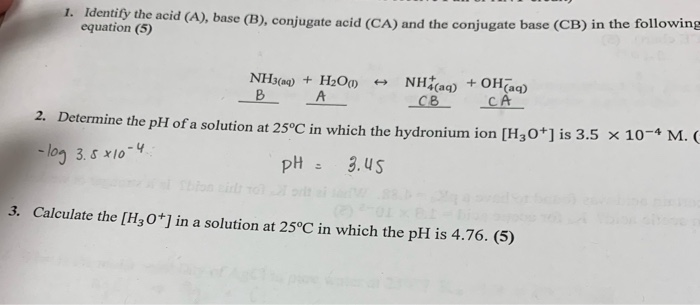1. Identify the acid (A), base (B), conjugate acid (CA) and the conjugate base (CB) in the following equation (5) NH3(aq) + H2O + NH(aq) + OH(aq) B CB CA 2. Determine the pH of a solution at 25°C in which the hydronium ion [H3O+] is 3.5 X 10 - log 3.5x10-4 - pH = 3.45 M. C 3. Calculate the [H3O+] in a solution at 25°C in which the pH is 4.76. (5)

• ### 16.2 Identify the acid-base conjugate pairs in each of the following reactions. (a) CH3COO- + HCN...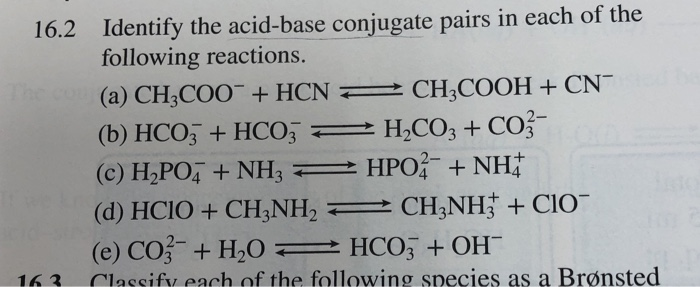16.2 Identify the acid-base conjugate pairs in each of the following reactions. (a) CH3COO- + HCN > CH4COOH + CNC (b) HCO3 + HCO3 = H2CO3 + CO3- (C) HAPO + NH3 HPO + NH (d) HCIO + CH3NH, 2 CH3NH+ + C10- (e) CO3- + H20 = HCO3 + OH 163 Classify each of the following species as a Brønsted

• ### Identify the Bronsted-Lowry acid, base, conjugate acid and the conjugate base of the following: CO32- CH3NH3...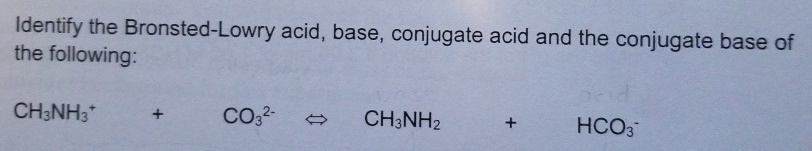Identify the Bronsted-Lowry acid, base, conjugate acid and the conjugate base of the following: CO32- CH3NH3 CH3NH2 НСО: +

Free Homework App